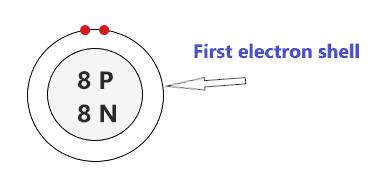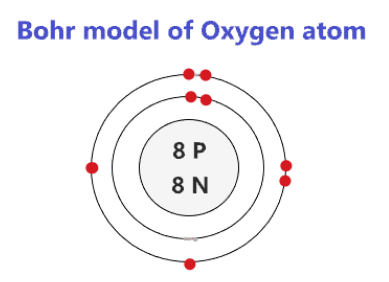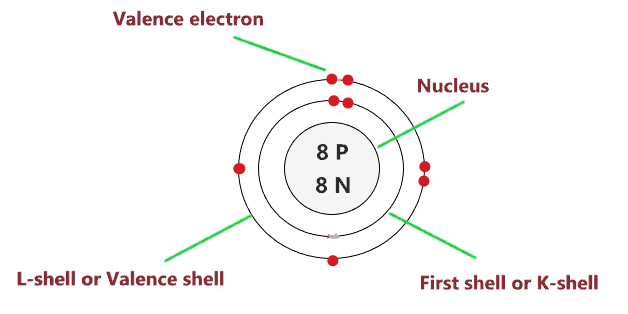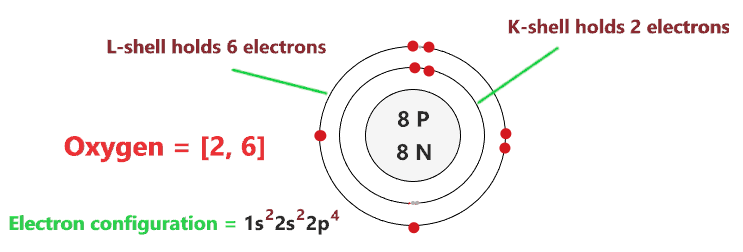# Bohr model of Oxygen atom - How to draw Oxygen(O) Bohr-Rutherford diagram?

Home  > Chemistry Article > Oxygen Bohr model

The Bohr Model of Oxygen(O) has a nucleus that contains 8 neutrons and 8 protons. This nucleus is surrounded by two-electron shells named K-shell and L-shell. The outermost shell in the Bohr diagram of Oxygen contains 6 electrons that also called valence electrons.

 Name Oxygen Bohr Model Number of neutrons 8 Number of protons 8 Number of electrons 8 Total electron shells 2 Electron in the First shell(K) 2 Electrons in the Second shell(L) 6 Total valence electrons in Oxygen 6
Page Contents

## How to draw Bohr Model of Oxygen(O)?

Bohr model describes the visual representation of orbiting electrons around the small nucleus. It used different electron shells such as K, L, M, N…so on. These shells hold a specific number of electrons, the electron shell which is closest to the nucleus has less energy and the electron shell which is farthest from the nucleus has more energy.

Bohr diagram is very interesting and easy to draw. Here, we will draw the Bohr diagram of the Oxygen atom with some simple steps.

## Steps to draw the Bohr Model of Oxygen atom

1. Find the number of protons, electrons, and neutrons in the Oxygen atom

Protons are the positively charged particles and neutrons are the uncharged particles, both these are constituents of the atom nuclei. Electrons are the negatively charged particles that orbit the nucleus of an atom

To find the number of protons an atom contains, just look at their atomic number.

If the atomic number of atom is 10, then proton will also be 10, if atomic number is 15, then proton will also be 15.

So, the atomic number for Oxygen is 8, hence, the number of protons in the Oxygen atom is also 8.Now, to determine the number of neutrons in an atom, use this formula.

⇒ Number of neutrons in atom = Atomic mass of the atom(rounded to the nearest whole number) – Number of proton in an atom

For example, An atom have 14.993 atomic mass and 10 protons.

Then, to find the number of neutron, round the atomic mass to the near whole number, so, atomic mass 14.993 round to 15.

= (15 – 10 protons) = 5 number of neutrons in the atom

Now, To get the number of neutrons in an Oxygen atom, look at its atomic mass which is 15.99 rounded to 16, and the number of protons in oxygen is 8.

∴ Hence, the number of neutrons in Oxygen atom = (16 – 8) = 8It should be noted that “The number of electrons in a neutral atom is equal to the number of protons”.

So, the Oxygen atom is neutral, hence, its number of electrons will be equal to the number of protons which is Eight as we already discussed.

⇒ The number of electrons in Oxygen atom = 8

⇒ The number of protons in Oxygen atom = 8

⇒ The number of neutrons in Oxygen atom = 8

2. Draw the nucleus of an atom

A nucleus is a dense and small region that contains the number of protons and neutrons of an atom.

In this step, we have to draw a small circle that consists of a number of protons and the number of neutrons of an Oxygen atom.3. Draw the First electron shell

“An electron shell may be thought of as an orbit followed by electrons around an atom’s nucleus.”

The first electron shell is also called the K-shell, this is the closest shell to the nucleus of an atom and can hold a maximum of two electrons.

As we know, the Oxygen atom has a total of 8 electrons. So, put two electrons from it, in the first shell, next to each other.We have successfully drawn the first shell of the Oxygen atom that can hold 2 electrons. As an Oxygen atom has a total of 8 electrons, and from 8 electrons we have used two electrons in the first shell.

∴ (8 – 2) = 6 electrons

Therefore, we are left with 6 electrons, let’s put them in the next shell of the Oxygen atom.

4. Draw the Second electron shell

The second shell also called the L-shell that can hold a maximum of 8 electrons. This shell is drawn after the first electron shell.

Since we already used two electrons in the first shell, now, we have to put the remaining 6 electrons in the second shell.

In the second electron shell, the electrons are added one at a time, starting from the top position then going in a clockwise direction.

In second shell, electrons are added one at a time in clockwise direction as a clock position – 12 o’clock, 3 o’clock, 6 o’clock, 9 o’clock positions.Once you place the electrons one at a time to each of the four sides(Top – Right – Bottom – Left], start pairing or doubling them.

So, we have to put 6 remaining electrons of the Oxygen atom in the second shell, hence, start from the top position, put electrons one at a time, and go in a clockwise direction(Top – Right – Bottom – Left). And, finally, start pairing.### Bohr model of an Oxygen atom

That’s all, this is our Bohr model of the Oxygen atom that contains 8 protons and 8 neutrons in the nucleus region, and 8 electrons are orbited around the nucleus, two electrons in the first shell, and six electrons in the second shell.

## Find Valence electron of Oxygen through its Bohr diagram

Valence electrons are found in the outermost shell of an atom and they can take participate in the formation of a chemical bond. These electrons have more energy compare to the inner shell electrons

From the Bohr diagram of an atom, we can easily find the number of valence electrons in an atom by looking at its outermost shell.

So, we have to find a valence electron in the Oxygen atom, for this, look at its Bohr diagram.

Bohr’s diagram of Oxygen has only two electron shells (K and L), the inner shell is K-shell and the outermost shell is L-shell.

Hence, the electrons found in the L-shell of the Oxygen atom are its valence electrons because it is the outermost shell that also called the valence shell.

The L-shell or outer shell of the Oxygen Bohr model contains 6 electrons, therefore, the number of valence electrons in the Oxygen atom is 6.## Electron dot diagram of the Oxygen atom

Electron dot diagram also called lewis structure which represents the valence electrons of atoms.

As, from the Bohr diagram of Oxygen, we got to know, it has 6 valence electrons.

So, just represent these 6 valence electrons around the Oxygen atom as a dot.## The Electron configuration of Oxygen

Electron configuration is the distribution of electrons of an atom or molecule in atomic or molecule orbitals”

Oxygen has an atomic number of 8 and it contains a total number of 8 electrons. From the Bohr model of Oxygen, we know, 2 electrons are in the K-shell and 6 electrons are in the L-shell.

So, based on the shell, the electronic configuration of the Oxygen atom is [2, 6].

Or the electronic configuration of Oxygen is 1s22s22p4 since it contains a total of 8 electrons, the first two-electron will go in 1s orbital, the next two in 2s orbital, and the remaining four electrons will go in 2p orbital.

S orbital can hold maximum of 2 electrons and P orbital can hold maximum of 6 electrons.## FAQ

### How many electron shells an Oxygen Bohr model contains?

Electron shell also called energy level, you can find the number of electron shells for an element by knowing its period number in the periodic table.

The elements or atoms in the first period of the periodic table have one energy level or one electron shell, same as, the elements in the second period have two energy levels or two-electron shells and so on…

So, the Oxygen atom belongs to the 2nd Period in the periodic table, hence, the number of electron shells for the Bohr model of Oxygen will be 2 (K-shell and L-shell).

### What is the outer shell of the Bohr diagram of the Oxygen atom?

The outermost shell also called the valence shell, which contains valence electrons of the atom.

According to the Bohr diagram of Oxygen, the outer shell is L-shell which contains 6 valence electrons.

## Properties of Oxygen

1. It appears colorless in gas form and pale blue in solid and liquid form.
2. It belongs to period 2, p-block, and group 16 (chalcogens).
3. Its electronegativity is 3.44.
4. It has a cubic crystal structure.
5. Oxygen is the third-most abundant element in the universe.

## Summary

• The Bohr model of Oxygen is drawn with only two electron shells, the first shell contains 2 electrons and the second shell contains 6 electrons.
• Oxygen is neutral and its atomic number is 8, hence, the number of protons and electrons available for its Bohr diagram is also 8.
• The number of neutrons for the Bohr diagram of Oxygen can be found by subtracting the number of protons from the atomic mass(rounded to the nearest whole).
• The electron configuration of Oxygen in terms of the shell is [2, 6], or in normal form, it is [He] 2s2 2p4.
##### Subscribe to Blog via Email

Join 2 other subscribers

Share it...